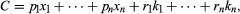# Theory of production | economics

## The production function

However much of a commodity a business firm produces, it endeavours to produce it as cheaply as possible. Taking the quality of the product and the prices of the productive factors as given, which is the usual situation, the firm’s task is to determine the cheapest combination of factors of production that can produce the desired output. This task is best understood in terms of what is called the production function, i.e., an equation that expresses the relationship between the quantities of factors employed and the amount of product obtained. It states the amount of product that can be obtained from each and every combination of factors. This relationship can be written mathematically as y = f (x1, x2, . . ., xn; k1, k2, . . ., km). Here, y denotes the quantity of output. The firm is presumed to use n variable factors of production; that is, factors like hourly paid production workers and raw materials, the quantities of which can be increased or decreased. In the formula the quantity of the first variable factor is denoted by x1 and so on. The firm is also presumed to use m fixed factors, or factors like fixed machinery, salaried staff, etc., the quantities of which cannot be varied readily or habitually. The available quantity of the first fixed factor is indicated in the formal by k1 and so on. The entire formula expresses the amount of output that results when specified quantities of factors are employed. It must be noted that though the quantities of the factors determine the quantity of output, the reverse is not true, and as a general rule there will be many combinations of productive factors that could be used to produce the same output. Finding the cheapest of these is the problem of cost minimization.

The cost of production is simply the sum of the costs of all of the various factors. It can be written: